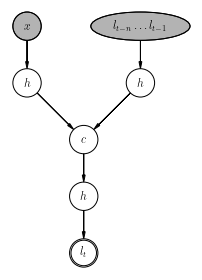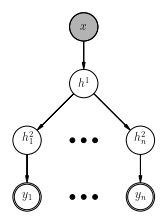# Plurals and Machine Learning

Using older machine learning models to conjugate English verbs produced rather silly results. These models performed at an acceptable level for many words, but when given nonsense words as an input these models would produce humorous conjugations. For example, we have:

VerbHuman Generated Past-TenseMachine Generated Past-Tense
mailmailedmembled
conflictconflictedconflafted
winkwinkedwok
quiverquiveredquess
satisfysatisfiedsedderded
smairfsmairfedsprurice
trilbtribledtreelilt
smeejsmeejedleefloag
frilgfrilgedfreezled

Naturally, my girlfriend and I found this hilarious. With the use of the XTAG database, we thought it would be fun to play around with a similar model to pluralize English nouns.

A jupyter notebook with all of the code from this investigation will be available on github under `leefloag.py`.

### Setup

The XTAG database is written in a flat ascii format, which doesn’t play nice with modern systems, so it needs some cleaning up.

``````def isplnoun(*line):
for entry in line:
if str(entry).isupper():
if re.match('N 3pl\$', str(entry)):
return True
return False
``````

This is a simple function which takes an entry from the XTAG database and checks each cell for the noun, plural tag, not counting the Genitive nouns.

``````raw = pd.read_csv('leefloag/morph_english.flat', sep='\t', lineterminator='\n',
names=['pl', 'blk', 'sg', 'pos1', 'pos2', 'pos3', 'pos4'])
raw = raw.values
clean = raw[[not x.startswith(';') for x in raw[:,0]], :]
clean = np.delete(clean, 1, axis=1)

mask = [isplnoun(*x) for x in clean]
clean = clean[:, :2]
clean = clean[~pd.isnull(clean).any(axis=1)]
clean = np.array([[re.sub('[^a-z]', '', x.strip().lower()), re.sub('[^a-z]','', y.strip().lower())] for x, y in clean])
``````

This code uses pandas’s super fast `read_csv()` function to import our file quickly and then just turn it into a numpy array for ease of generalization. We remove the comment lines from the beginning, and then turn our `isplnoun()` function into a boolean mask to remove all the lines that aren’t plural non-genitive nouns. Then cut off the part-of-speech information since we don’t need that anymore. Then we remove lines with null inputs.

### Vectorization

We’re going to need a vector encoding of each of our words. We think that the vectorization ought to preserve order, so we are just going to cipher our text as integers. We’ll scan through the whole corpus and get a set of all the characters used, and then we’ll use that to create a simple mapping and inverse mapping. We’ll use `'?'` as our end-of-frame character.

``````charset = set()
for x in clean.flatten():
for y in x:
mapping = {c: i for i, c in enumerate(charset)}
mapping['?'] = len(mapping)
invmap = {i: c for c, i in mapping.items()}
``````

Of course we’ll also need functions to turn those lists of words into lists of vectors.

``````def map_encode(*words, code=mapping):
output = []
lens = np.vectorize(len)(words)
maxlen = max(lens)
for word in words:
xpand = list(word)
mapped = [code[y] for y in xpand]
output.append(mapped)
return output

def map_decode(*ctext, code=invmap): # The ctest stands for 'ciphertext'
output = []
for word in ctext:
dcoded = [code[x] for x in word]
dcoded = ''.join(dcoded).strip('?')
output.append(dcoded)
return output
``````

So running `map_encode(*['coen'])` returns something like: `[[16, 25, 3, 1]]` and `map_encode(*['coen', 'needell'])` returns something like : `[[16, 25, 3, 1, 26, 26, 26], [1, 3, 3, 10, 3, 24, 24]]`. You’ll notice a lot of ‘3’s because I have a boatload of ’e’s in my name, and the map generation uses a set, so the order is not the same as the order of the alphabet. Now, finally, we transform our inputs and outputs.

``````invecs = map_encode(*clean.transpose())
outvecs = map_encode(*clean.transpose())
``````

### Plurals and Letter-by-Letter Models

A letter-by-letter model works with two inputs, the singular word, and the last n letters that the model has guessed. In the below diagram, x represents the singular word, and the plural word is l. The subscript on l represents the tth letter in the word. h represents a hidden layer, and c represents a concatenate layer, or a layer which serves to merge two layers. For our purposes, each of these layers are densely connected.For example, let’s say we input the word ‘coen’. In that case x is ‘coen’, and we’ll run the model until the output lt is the end of frame character `'?'`. For this illustration we’ll use n = 3, but in the real model I got good results with n = 7. Assuming a correct model, when we input ‘coen’, we run the first round with `x = 'coen'` and `l = '???'`. We run the model once with these inputs, and it tells us that l0 = `'c'`. Now we run it again with `l = '??c'`, it tells us that l1 = `'o'`. Again and we get `'e'`, then `'n'`, then `'s'`, and then `'?'`, leaving us with a final answer of `l = 'coens'`.

Here’s the code I used.

First, some data wrangling. We have to take our database that’s organized like:

InputOutput
JessicaJessicae
MooseMeese
HouseHice

We need one that instead looks like:

Input 1Input 2Output
Jessicaessi
Jessicassic
Mooseeese
Mooseese?
House??hi
House?hic
``````def genngrams(word, n):
wordpad = '?' * (n) + word + '?' * (n-1)
ngrams = zip(*[wordpad[i:] for i in range(n + 1)])
for j in ngrams:
gram = j[:n]
nextchar = j[-1]
yield ''.join(gram), nextchar

lblins = []
lblprevs = []
lblouts = []

for o, i in clean:
for gram3, nextchar in genngrams(o, 7):
lblins.append(i)
lblprevs.append(gram3)
lblouts.append(nextchar)

lblins = tf.keras.utils.to_categorical(np.array(map_encode(*lblins)))
lblprevs = tf.keras.utils.to_categorical(np.array(map_encode(*lblprevs, l=7)))
lblouts = tf.keras.utils.to_categorical(np.array(map_encode(*lblouts, l=1)))
``````

Most of this cleaning is self-explanatory. We need a function to split a word into a list of all n-clusters of letters, and the next letter, enter `genngrams()`. We just run this on every word in the corpus, and record them in the variables `lblins`, `lblprevs`, and `lblouts`. Then we turn them into keras-friendly categoricals. These replace a list of integers with a boolean array.

Now let’s build a model.

``````minput = tf.keras.layers.Input(shape=(lblins.shape, lblins.shape), name='main_input')
mf = tf.keras.layers.Flatten()(minput)
mh = tf.keras.layers.Dense(64, activation='relu')(mf)

pinput = tf.keras.layers.Input(shape=(lblprevs.shape, lblprevs.shape), name='prev_output')
pf = tf.keras.layers.Flatten()(pinput)
ph = tf.keras.layers.Dense(64, activation='relu')(pf)

f = tf.keras.layers.concatenate([ph, mh])
``````

This forms the top half of the diagram from before. We create the input (greyed) nodes, and the hidden networks below them. Then concatenate the two. Now let’s make the bottom half.

``````h = tf.keras.layers.Dense(512, activation='relu')(f)
for _ in range(10):
h = tf.keras.layers.Dense(512, activation='relu')(h)

outlayer = tf.keras.layers.Dense(27, activation='softmax')(h)
``````

The hidden network below the concatenation layer is made with this for loop, we have a fairly deep one. Then we follow that up with a single categorical output. That tells us the output letter.

``````lblnlayer = tf.keras.models.Model(inputs=[minput, pinput], outputs=[outlayer])

lblnlayer.fit([lblins, lblprevs], lblouts, epochs=20, validation_split=.1)
``````

Then we compile and fit the model. Since this has a simpler output, and so many more data points associated with it, we can use a validation split to better understand the fitting and loss curves. We’ll use standard categorical loss, and the AdaM optimizer.

InputOutput
coencc
jessicajoeiecas
mousemm
smeejstt
doordd
deerdd
moosemm
jeffjv
gerpgorkggtdcors
leefloagllrluoags
elfec
childcc
focusff
torusttt
househh
frilgffrrgs
walrusww

Wow, that’s… awful.

### Plurals and All-At-Once Models

An all-at-once model works by taking the singular word as an input, pushes it through a hidden network, and then produces an output letter for each possible letter (up to a maximum length, which is determined by the length of the longest word in the corpus). In the diagram below, x is the singular version of the word, the hij are hidden layers, and yt are the tth letter in the plural word. This is conceptually a lot simpler than the letter-by-letter model, although producing all-at-once models in tensorflow can be a little more complicated.Here’s the code I used:

First we need to do a little bit more pre-processing to get our data to play nice with tensorflow.

``````in_train = tf.keras.utils.to_categorical(np.array(map_encode(*clean.transpose())))
out_train = tf.keras.utils.to_categorical(np.array(map_encode(*clean.transpose())))
better_outs = [out_train[:, x, :] for x in range(out_train.shape)]
``````

First, we make the input layer, x on the diagram.

``````minput = tf.keras.layers.Input(shape=(in_train.shape, in_train.shape), name='main_input')
f = tf.keras.layers.Flatten()(minput)
h = tf.keras.layers.Dense(512, activation='relu')(f)

h = tf.keras.layers.Dense(512, activation='relu')(h)
h = tf.keras.layers.Dense(512, activation='relu')(h)
``````

Now for making the output layers, everything below the split on the diagram.

``````out_layers = []
for letter in range(in_train.shape):
hout = tf.keras.layers.Dense(128, activation='relu')(h)
hout = tf.keras.layers.Dense(128, activation='relu')(hout)
out_layers.append(tf.keras.layers.Dense(in_train.shape, activation='softmax')(hout))
``````

Finally the tensorflow compilation. We’re using `categorical_crossentropy` as the loss, because the model works by assigning each letter to a category, with the categories being all of the letters, plus the end of frame character `'?'`. We’re using the AdaM optimizer, half because I like it, and half because it’s fairly popular for natural language applications. We’re also having the model record the `categorical_accuracy` so we have a human-readable metric of success.

``````
tlayermodel = tf.keras.models.Model(inputs=[minput], outputs=out_layers)

tlayermodel.fit(in_train, better_outs, epochs=100)
``````

Here’s a table of selected results. On my laptop, with an Nvidia 1070 GPU, this took about an hour to train for 100 epochs. I suspect somewhere around 40 epochs will suffice. Here is a table of results.

InputOutputMy Pluralization
coencoenscoens
jessicajessicasjessicae
mousemicemice
smeejsmeetssmeeges (soft g)
doordoorsdoors
deerdeersesdeer
moosemoosesmoosuch
jeffjeffsjeffs
gerpgorkgerpgorksgerpgorks
leefloagleefloagsleefloags
elfelveselves
childchildrenchildren
focusfocifoci
torustoritori
househousehouses
frilgfrilgsfrilges
walruswalruswalruses/walrii

Pretty good results. Some of them are even funny, like ‘deerses’. We seem to have accidentally made a good pluralization model. But wait, maybe it’s just memorized all the real words, and gets close enough for my made up ones, what happens if we feed it really weird words?

InputOutput
cuddlewugcuddlewugs
wugwughi
buddlesnubuddlesnu
bunderkindbunderkinds
goofusgoofuses
terminatorterminators
attaccbirdattaccbirds
hawkhawks
claudioclaudios
steviestevies
knickersknickerses
bunchesrunchbunchesrunche
bunchacrunchbuncsacrunies
anqianqis
attorneygeneralahtornebmeners

Now there’s some funny results. But sadly, none of them really match smeej -> leefloag.